TitleCollege Algebra
Tutorial 21: Absolute Value
Equations

WTAMU > Virtual Math Lab > College AlgebraLearning Objectives

 After completing this tutorial, you should be able to: Solve absolute value equations.Introduction

 In this tutorial, I will be stepping you through how to solve equations that have absolute values in them.  We will first go over the definition of absolute value and then use that to help us solve our absolute value equations.  You will find that when you have an absolute value expression set equal to a positive number, you will end up with two equations that you will need to solve to get your solutions.  Since the absolute value of any number other than zero is positive, it is not permissible to set an absolute value expression equal to a negative number.  So, if your absolute value expression is set equal to a negative number, then you will have no solution.  The two main things that you need to know from your past that will help you work the types of problems in this tutorial is how to solve linear and quadratic equations.  If you need a review on solving linear equations, feel free to go to Tutorial 14:  Linear Equations in One Variable.  If you need a review on solving quadratic equations, feel free to go to Tutorial 17:  Quadratic Equations.  Ready, set, GO!!!Tutorial

 Absolute Value

 A lot of people know that when you take the absolute value of a number the answer is positive, but do you know why?  Let's find out: The absolute value measures the DISTANCE a number is away from the origin (zero) on the number line.  No matter if the number is to the left (negative) or right (positive) of zero on the number line, the DISTANCE it is away from zero is going to be positive. Hence, the absolute value is always positive (or zero if you are taking the absolute value of 0).Example 1: What two numbers have an absolute value of 7?

 If you said 7 and -7, you are correct - good for you.  Now, I want to explain the thought behind it because this is going to help us to understand how to solve absolute value equations.  I really want to emphasize the fact that there are two numbers that are the same distance away from the origin, the positive number and its opposite.  The thought behind this is there are two places on the number line that are 7 units away from zero - both 7 and -7.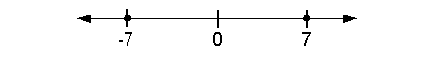Solving an Absolute Value Equation

 Step 1:  Use the definition of absolute value to set up the equation without absolute values.

 If d is POSITIVE and |x| = d, then x = d    OR    x = -d (two equations are set up) If d is NEGATIVE and |x| = d, then No solution  This is because distance (d)  can not be negative.

 Step 2: Solve the equations set up in step 1.

 The equations in this tutorial will lead to either a linear or a quadratic equation. If you need a review on solving linear equations, feel free to go to Tutorial 14: Linear Equations in One Variable.  If you need a review on solving quadratic equations, feel free to go to Tutorial 17:  Quadratic Equations.Example 2: Solve the absolute value equation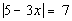.

 We need to think about what value(s) are 7 units away from zero on the number line. Using the definition of absolute value, there will be two equations that we will need to set up to get rid of the absolute value because there are two places on the number line that are 7 units away from zero: 7 and -7.

 First equation: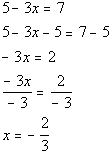*Setting inside linear expression = to 7 *Inv. of add. 5 is sub. 5   *Inv. of mult. by -3 is div. by -3

 Second equation: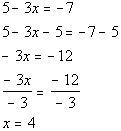*Setting inside linear expression = to -7 *Inv. of add. 5 is sub. 5   *Inv. of mult. by -3 is div. by -3

 When we plug -2/3 in for x, we end up with the absolute value of 7 which is 7.  When we plug 4 in for x, we end up with the absolute value of -7 which is also 7. If you would have only come up with an answer of x = -2/3, you would not have gotten all solutions to this problem. Again, it is important to note that we are using the definition of absolute value to set the two equations up.  Once you apply the definition and set it up without the absolute value, you just solve the linear equation as shown in Tutorial 14: Linear Equations in One Variable.  There are two solutions to this absolute value equation:  -2/3 and 4.Example 3: Solve the absolute value equation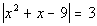.

 We need to think about what value(s) are 3 units away from zero on the number line. Using the definition of absolute value, there will be two equations that we will need to set up to get rid of the absolute value because there are two places on the number line that are 3 units away from zero: 3 and -3.

 First equation: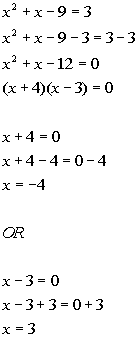*Setting inside quadratic expression = to 3   *Quad. eq. in standard form *Factor the trinomial *Use Zero-Product Principle *Set 1st factor = 0 and solve             *Set 2nd factor = 0 and solve

 Second equation: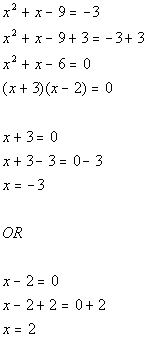*Setting inside quadratic expression = to -3   *Quad. eq. in standard form *Factor the trinomial *Use Zero-Product Principle *Set 1st factor = 0 and solve             *Set 2nd factor = 0 and solve

 When we plug -4 and 3 in for x, we end up with the absolute value of 3 which is 3.  When we plug -3 and 2 in for x, we end up with the absolute value of -3 which is also 3. If you would have only come up with an answer of x = -4 and 3, you would not have gotten all solutions to this problem. Again, it is important to note that we are using the definition of absolute value to set the two equations up.  Once you apply the definition and set it up without the absolute value, you just solve the quadratic equation as shown in Tutorial 17:  Quadratic Equations.  There is are four solutions to this absolute value equation: -4, 3, -3, and 2.Example 4: Solve the absolute value equation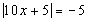.

 Be careful on this one.  It is very tempting to set this up the same way we did example 2 or 3 above, with two solutions.  However, note that the absolute value is set equal to a negative number. There is no value of x that we can plug in that will be a solution because when we take the absolute value of the left side it will always be positive or zero, NEVER negative. Answer: No solution.Example 5: Solve the absolute value equation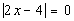.

 We need to think about what value(s) are 0 units away from zero on the number line. Using the definition of absolute value, there will be only one equation that we will need to set up to get rid of the absolute value, because there is only one place on the number line that is 0 units away from zero: 0.

 First equation: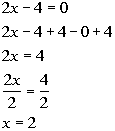*Setting inside linear expression = to 0 *Inv. of sub. 4 is add 4   *Inv. of mult. by 2 is div. by 2

 When we plug 2 in for x, we end up with the absolute value of 0 which is 0.  Again, it is important to note that we are using the definition of absolute value to set the equation up.  Once you apply the definition and set it up without the absolute value, you just solve the linear equation as shown in Tutorial 14: Linear Equations in One Variable.  There is only one solution to this absolute value equation: 2.Practice Problems

 These are practice problems to help bring you to the next level.  It will allow you to check and see if you have an understanding of these types of problems. Math works just like anything else, if you want to get good at it, then you need to practice it.  Even the best athletes and musicians had help along the way and lots of practice, practice, practice, to get good at their sport or instrument.  In fact there is no such thing as too much practice. To get the most out of these, you should work the problem out on your own and then check your answer by clicking on the link for the answer/discussion for that  problem.  At the link you will find the answer as well as any steps that went into finding that answer.Practice Problems 1a - 1c: Solve each absolute value equation.

 1a.(answer/discussion to 1a) 1b.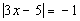(answer/discussion to 1b)

 1c.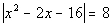(answer/discussion to 1c)Need Extra Help on these Topics?

The following is a webpage that can assist you in the topics that were covered on this page:

 http://www.sosmath.com/algebra/solve/solve0/solve0.html#absolute Only look at problem 1.  Problem 1 at this webpage helps you with solving absolute value equations.

Go to Get Help Outside the Classroom found in Tutorial 1: How to Succeed in a Math Class for some more suggestions.

WTAMU > Virtual Math Lab > College Algebra

Videos at this site were created and produced by Kim Seward and Virginia Williams Trice.
Last revised on Dec. 16, 2009 by Kim Seward.